Courses

# RCC (Concrete Structures) - 2

## 20 Questions MCQ Test GATE Civil Engineering (CE) 2022 Mock Test Series | RCC (Concrete Structures) - 2

Description
This mock test of RCC (Concrete Structures) - 2 for GATE helps you for every GATE entrance exam. This contains 20 Multiple Choice Questions for GATE RCC (Concrete Structures) - 2 (mcq) to study with solutions a complete question bank. The solved questions answers in this RCC (Concrete Structures) - 2 quiz give you a good mix of easy questions and tough questions. GATE students definitely take this RCC (Concrete Structures) - 2 exercise for a better result in the exam. You can find other RCC (Concrete Structures) - 2 extra questions, long questions & short questions for GATE on EduRev as well by searching above.
QUESTION: 1

Solution:
QUESTION: 2

### In case of simply supported slabs spanning in two directions, the maximum permissible value of span/depth ratio is ______

Solution:

SPAN to OVERALL DEPTH RATIO
for SLABS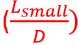For Live Loads upto 3kN/m2 for Fe250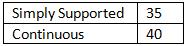For Fe415 multiply the above values with 0.8

QUESTION: 3

### The maximum depth of the neutral axis for a beam with d as the effective depth, in the limit state method of design for Fe 415 steel, is

Solution: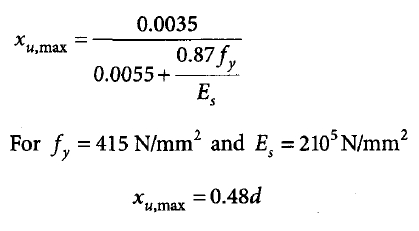QUESTION: 4

In the limit state design method, the moment of resistance for a balanced section using M20 grade concrete and HYSD steel of grade Fe 415 is given by Mµ, lim = kbd2 . What is the value of K?

Solution: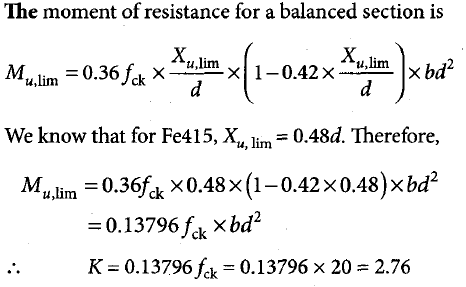QUESTION: 5

What is the adoptable maximum spacing between vertical stirrups in an RCC beam of rectangular cross-section having an effective depth of 300 mm?

Solution: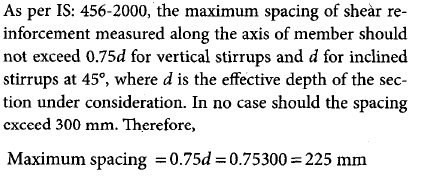QUESTION: 6

A simply supported RC beam having clear span of 5 m and support width of 300 mm has the crosssection as shown in the figure below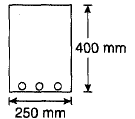What is the effective span of the beam as per IS: 456?

Solution: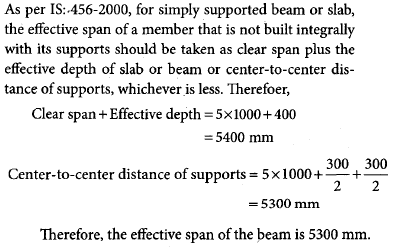QUESTION: 7

The main reinforcement of an RC slab consists of 10 mm bars at 100 mm spacing. If it is desired to replace the 10 mm bars by 12 mm bars, then the spacing of 12 mm bars should be ___________ mm.

Solution: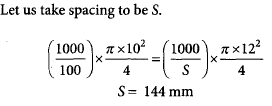QUESTION: 8

The minimum strain at failure in the tensile reinforcement (Fy = 400 MPa) of RCC beam as per the limit state method is

Solution: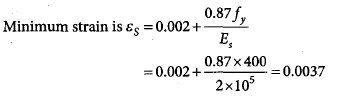QUESTION: 9

Column-I gives a list of test methods of evaluating the properties of concrete and column-II gives the list of properties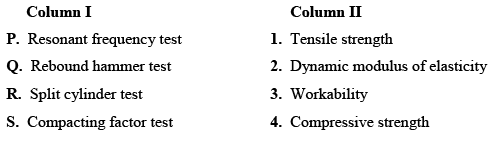The correct match of the test with the property is

Solution:
QUESTION: 10

Which one of the following is categorized as the long term loss of prestress in a prestressed concrete member:

Solution:
QUESTION: 11

Consider the following statements:

Curing of concrete is necessary because

1. Concrete needs more water for chemical reaction

2. It is necessary to protect the water initially mixed in concrete from being lost during evaporation

3. Penetration of surrounding water increases the strength of concrete

Which of the statement/s given above is/are correct?

Solution:
QUESTION: 12

At the limit state of collapse, an RC beam is subjected to flexural moment 200 kNm, shear force 20 kN, and torque 9 kNm. The beam is 300 mm wide and has a gross depth of 425 mm, with an effective cover of 25mm. The equivalent nominal shear stress ( τve ) as calculated using the design code turns out to be lesser than the design shear strength ( τc ) of the concrete.

Q.The equivalent shear force (Vc ) is

Solution: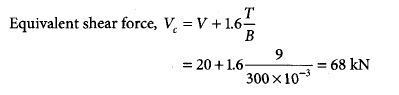QUESTION: 13

At the limit state of collapse, an RC beam is subjected to flexural moment 200 kNm, shear force 20 kN, and torque 9 kNm. The beam is 300 mm wide and has a gross depth of 425 mm, with an effective cover of 25mm. The equivalent nominal shear stress ( τve ) as calculated using the design code turns out to be lesser than the design shear strength ( τc ) of the concrete.

Q.The equivalent flexural moment (Mel ) for designing the longitudinal tension steel is

Solution: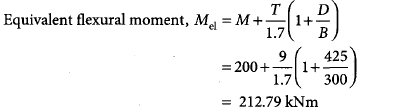QUESTION: 14

Assume a straight line instead of parabola for the stress-strain curve of concrete is follows and the partial factor of safety as 1.0.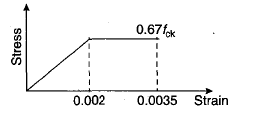A rectangular under-reinforced concrete section of 300 mm width and 500 mm effective depth is reinforced with three bars of grade Fe415, each of 16 mm diameter. The concrete mix is M20

Q.The depth of the neutral axis from the compression fiber is

Solution:QUESTION: 15

Assume a straight line instead of parabola for the stress-strain curve of concrete is follows and the partial factor of safety as 1.0.A rectangular under-reinforced concrete section of 300 mm width and 500 mm effective depth is reinforced with three bars of grade Fe415, each of 16 mm diameter. The concrete mix is M20

Q.The depth of the neutral axis obtained as per IS: 456-2000 differs from the depth of the neutral axis obtained in Q.14by

Solution: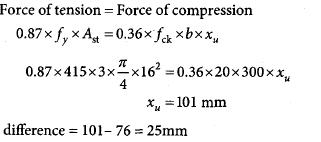QUESTION: 16

In the design of beams for the limit state of collapse in flexure as per IS: 456-2000, let the maximum strain in concrete be limited to 0.0025 (in place of 0.0035). For this situation, consider a rectangular beam section with breadth as 250 mm, effective depth as 350 mm, area of tension steel as 1500 mm2, and characteristics strengths of concrete and steel as 30 MPa and 250 MPa respectively

Q.The depth of the neutral axis for the balanced failure is

Solution: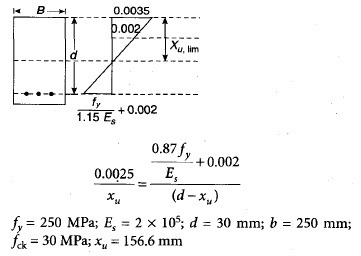QUESTION: 17

In the design of beams for the limit state of collapse in flexure as per IS: 456-2000, let the maximum strain in concrete be limited to 0.0025 (in place of 0.0035). For this situation, consider a rectangular beam section with breadth as 250 mm, effective depth as 350 mm, area of tension steel as 1500 mm2, and characteristics strengths of concrete and steel as 30 MPa and 250 MPa respectively

Q.At the limiting state of collapse in flexure, the force acting on the compression zone of the section is

Solution: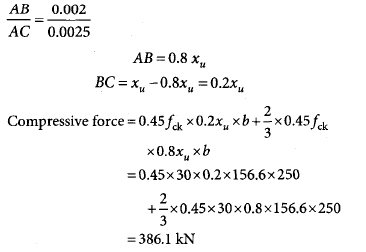QUESTION: 18

A doubly reinforced rectangular concrete beam has a width of 300 mm and an effective depth of 500 mm. The beam is reinforced with 2200 mm2 of steel in tension and 628 mm2 of steel in compression. The effective cover for compression steel is 50 mm. Assume that both tension and compression steel yield. The grades of concrete and steel used are M20 and Fe250, respectively. The stress lock parameters (rounded off to first two decimal places) for concrete should be as per IS: 456-2000.

Q.The depth of the neutral axis is

Solution: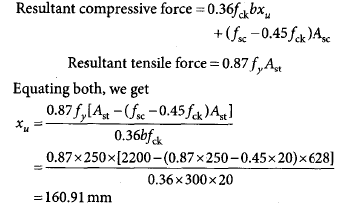QUESTION: 19

A doubly reinforced rectangular concrete beam has a width of 300 mm and an effective depth of 500 mm. The beam is reinforced with 2200 mm2 of steel in tension and 628 mm2 of steel in compression. The effective cover for compression steel is 50 mm. Assume that both tension and compression steel yield. The grades of concrete and steel used are M20 and Fe250, respectively. The stress lock parameters (rounded off to first two decimal places) for concrete should be as per IS: 456-2000.

Q.The moment of resistance of the section is

Solution: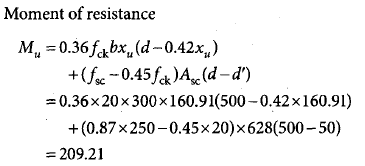QUESTION: 20

onsider a bar of diameter D embedded in a large concrete block as shown in the adjoining figure, with a pullout force P being applied. Let σb andσst be the bond strength (between the bar and concrete) and the tensile strength of the bar, respectively. If the block is held in position and it is assumed that the material of the block does not fail, which of the following option represents the maximum value of P?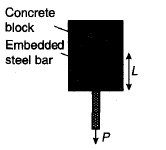Solution: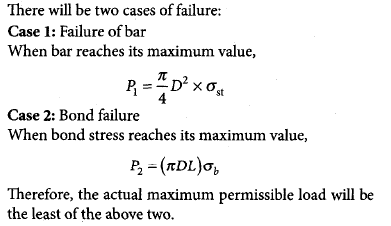• RCC - 2